Courses

# Multiple Choice Questions- Linear Equations in One Variable Class 8 Notes | EduRev

## Class 8 Mathematics by Full Circle

Created by: Full Circle

## Class 8 : Multiple Choice Questions- Linear Equations in One Variable Class 8 Notes | EduRev

The document Multiple Choice Questions- Linear Equations in One Variable Class 8 Notes | EduRev is a part of the Class 8 Course Class 8 Mathematics by Full Circle.
All you need of Class 8 at this link: Class 8

1. In the equation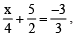transposing 5/2 to RHS, we get:

(i)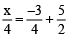(ii)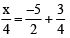(iii)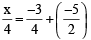(iv) none of these

2. In the equation 3x = 4 – x , transposing (–x) to LHS, we get:

(i) 3x – x = 4
(ii) 3x + x = 4
(iii) –3x + x = 4
(iv) –3x – x = 4

3.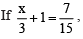then which of the following correct?

(i)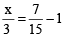(ii)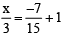(iii)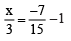(iv) none of these

4. If 7x + 15 = 50, then which of the following is the root of the equation?

(i) –5
(ii) 65/7
(iii) 5

(iv) 1/5

5. If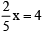then which of the following is the value of x?

(i) 10
(ii) –10

(iii) -8/5
(iv) 8/5

6. In the sum of two consecutive numbers is 71 and one of them being x, then which of the following is the other number?

(i) x + (x + 1) = 71
(ii) x + (x + 2) = 71
(iii) x + x = 71
(iv) none of these

7. Two years ago my age was ‘x’ years 5 years ago my age was?

(i) (x + 7) years
(ii) (x – 2 – 5) years
(iii) (x – 5) years
(iv) (x – 3) years

8. 10 years ago I was ‘x’ years old. After 10 years, my age will be:

(i) (x + 20) years
(ii) (x – 20) years
(iii) (x + 10) years
(iv) (x –10) years

9. If the difference of two consecutive numbers is 15 and the greater of them is x then the smaller number is:

(i) 16
(ii) 14
(iii) 8
(iv) 7

10. If ‘x’ is an even number, then which of the following is the next odd number is?

(i) x + 1
(ii) x + 2
(iii) x – 1
(iv) x – 2

1. (iii)

2. (ii)

3. (i)

4. (iii)

5. (i)

6. (i)

7. (iv)

8. (i)

9. (iv)

10. (i)

93 docs|16 tests

,

,

,

,

,

,

,

,

,

,

,

,

,

,

,

,

,

,

,

,

,

;